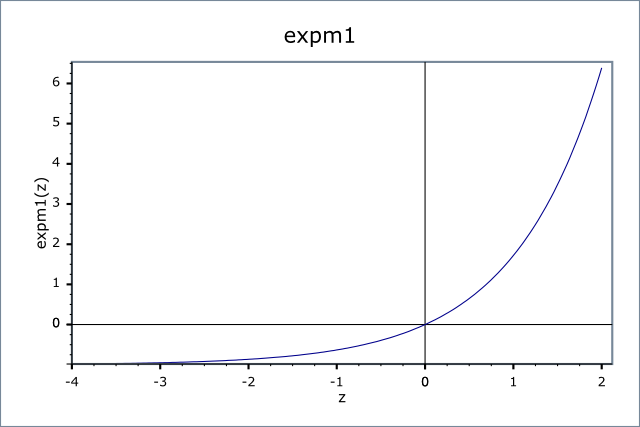#Boost C++ Libraries

...one of the most highly regarded and expertly designed C++ library projects in the world.

This is the documentation for an old version of boost. Click here for the latest Boost documentation.

#### expm1

```#include <boost/math/special_functions/expm1.hpp>
```

```namespace boost{ namespace math{

template <class T>
calculated-result-type expm1(T x);

template <class T, class Policy>
calculated-result-type expm1(T x, const Policy&);

}} // namespaces
```

Returns ex - 1.

The return type of this function is computed using the result type calculation rules: the return is `double` when x is an integer type and T otherwise.

The final Policy argument is optional and can be used to control the behaviour of the function: how it handles errors, what level of precision to use etc. Refer to the policy documentation for more details.

For small x, then `ex` is very close to 1, as a result calculating `ex - 1` results in catastrophic cancellation errors when x is small. `expm1` calculates `ex - 1` using rational approximations (for up to 128-bit long doubles), otherwise via a series expansion when x is small (giving an accuracy of less than 2ɛ).

Finally when BOOST_HAS_EXPM1 is defined then the `float/double/long double` specializations of this template simply forward to the platform's native (POSIX) implementation of this function.

The following graph illustrates the behaviour of expm1:##### Accuracy

For built in floating point types `expm1` should have approximately 1 epsilon accuracy.

##### Testing

A mixture of spot test sanity checks, and random high precision test values calculated using NTL::RR at 1000-bit precision.